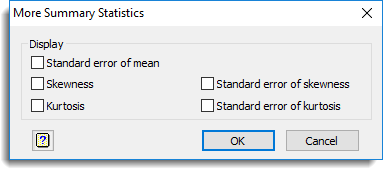1. Home
2. More Summary Statistics

# More Summary Statistics

Provides additional statistics to be calculated for summarizing group data.## Options

You can choose one or more of the following statistics to be calculated:

 Standard error of mean Standard error of skewness Skewness Standard error of kurtosis Kurtosis

## Method

 Unweighted Weighted Skewness Σ(x – (Σx/n))3 / ( Σ(x – (Σx/n))2 )3/2 Σ w (x – (Σwx/Σw))3 / ( Σ w (x – (Σwx/Σw))2 )3/2 Kurtosis Σ(x – (Σx/n))4 / ( Σ(x – (Σx/n))2 )2 – 3 Σ w (x – (Σwx/Σw))4 / Σ w (x – (Σwx/Σw))2 )2 – 3 s.e. skewness √( { 6n * (n-1) } / { (n-2) * (n+1) * (n+3) } ) √( { 6Σw * (Σw – 1) } / { (Σw – 2) * (Σw + 1) *     (Σw + 3) } ) (x not missing) s.e. kurtosis √( { 24 * n * (n-1)2 } / { (n-2) * (n-3) * (n+5) *   (n+3) } ) √( { 24 * Σw * (Σw – 1)2 } / { (Σw – 2) * (Σw – 3) *     (Σw + 5) * (Σw + 3) } ) (x not missing)